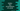# Python program to find the circumference of a circle## Python program to find the circumference of a circle :

In this tutorial, we will learn how to find the circumference of a circle in python 3. The circumference is the total distance around a circle. The distance around a rectangle or square is called perimeter. For a circle, it is called circumference.

The circumference of a circle is the product of ‘Pi’ and its diameter. If we draw a line through the centre of the circle from one end to the other end, it is called a diameter. The diameter is equal to two times the radius of a circle. If we draw a straight line from the centre to any point on the circumference of a circle, it is called a radius.

We can use the formula ‘2 * Pi * radius’ to calculate the circumference of a circle, where ‘radius’ is the radius for that circle. So, we need only the value of the radius to calculate the circumference.

Our program will take the radius value as an input from the user. The user will enter the radius, it will calculate the circumference and print out the result.

Let me quickly show you the algorithm that we are going to use in the program :

### The algorithm to find out the circumference of a circle :

The basic algorithm looks like as below :

1. Take the radius as an input from the user. Store the radius value in a variable. If you want, you can also take the diameter from the user as an input but the other formula is used widely.
2. Calculate the circumference of the circle by using the formula mentioned above. We can use one constant to store the value of ‘Pi’, but we can also use the ‘math’ module. ‘math’ module is an inbuilt module with lots of useful constants and formulae. In this example, I will show you how to use ‘Pi’ using the ‘math’ module.
3. Find out the circumference and print out the result to the user.

### Python program :

``````import math

radius = float(input("Enter the radius of the circle : "))

circumference = 2 * math.pi * radius

print("Circumference of the circle is : %.2f" % circumference)``````

You can also download this program from here.

### Explanation :

1. First, take the radius as an input from the user. The radius is a floating value. So, we are using ‘float(input())‘. input() method is used to read the user input value. This value is of string type. We are wrapping this value with ‘float()’ to get the float type.
2. Calculate the circumference. The value of Pi is taking from the ‘math‘ module of python. ‘math.pi‘ gives the value of pi. Note that we are importing this module at the start of the program. If you don’t import this module, it will throw you one exception.
3. Print the value of the circumference. The value is formatted up to two decimal places using %.2f.

### Conclusion :

In this tutorial, we have learned how to find out the circumference of a circle programmatically in python. We have learned how to use the math module and how to use the value of ‘Pi’ using the math module. Try to run the program and drop one comment below if you have any queries.